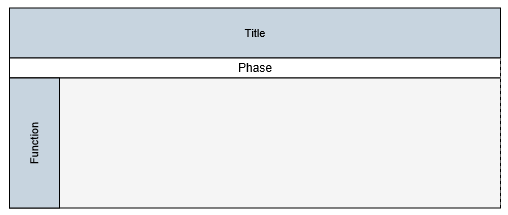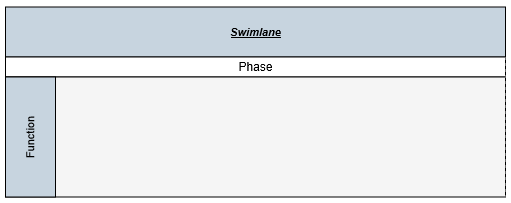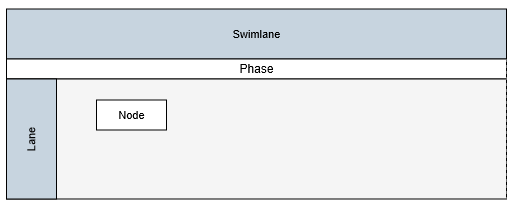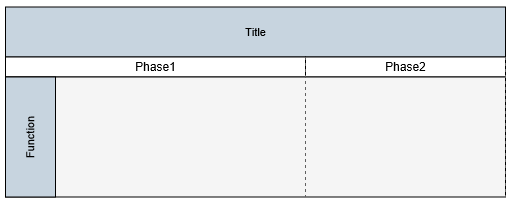# Swim lane

19 Jul 201923 minutes to read

Swim-lane Diagrams are typically used to visualize the relationship between a business process and the department responsible for it by focusing on the logical relationships between activities. Swimlanes may be arranged either horizontally or vertically.

##Initialize the Diagram model
Create a diagram properties model element that can be used to initialize the diagram widget as shown below.

• ASPX-CS
• ``````//Initialize the Diagram Model
<ej:Diagram ID="Diagram" runat="server" Height="700px" Width="900px">
</ej:Diagram>``````

## Create a swimlane

To create a swimlane, you need to use the `Swimlane` class. By default, the swimlanes are arranged vertically. You can change that with the `Orientation` property of swimlane.

The following code example illustrates how to define a swimlane object.

• ASPX-CS
• ``````<ej:DiagramSwimlane Name="Swimlane" Orientation="horizontal" OffsetX="400" OffsetY="200" Height="100" Width="700 ">
</ej:DiagramSwimlane>``````

Adding a swimlane to the Diagram is same as adding a node. You can add either through the `Nodes` collection or through the client side method `add`. You can also drag and drop a swimlane from symbol palette.

The following code example illustrates how to add a swimlane to the Diagram through `Nodes` collection.

• ASPX-CS
• ``````<!-- Add the swimlane in to the Nodes collection -->
<ej:Diagram ID="Diagram" runat="server" Height="700px" Width="900px">
<Nodes>
<ej:DiagramSwimlane Name="Swimlane" Orientation="horizontal" OffsetX="400" OffsetY="200" Height="100" Width="700 ">
</ej:DiagramSwimlane>
</Nodes>
</ej:Diagram>``````Swimlane allows to define a header to textually describe it. The `Header` property of swimlane allows you to define its textual description(`Text`) and to customize its appearance. The following code example illustrates how to define swimlane header.

• ASPX-CS
• ``````<ej:Diagram ID="Diagram" runat="server" Height="700px" Width="900px">
<%--Add the swimlane in to the Nodes collection--%>
<Nodes>
<%--Changes the orientation,position and size--%>
<ej:DiagramSwimlane Name="Swimlane" Orientation="horizontal" OffsetX="400" OffsetY="200" Height="100" Width="700 ">
<Header Text="Swimlane" Height="50" FillColor="#C7D4DF" FontColor="black" FontSize="11" FontFamily="Arial" Italic="true" Bold="true" TextDecoration="Underline">
</ej:DiagramSwimlane>
</Nodes>
</ej:Diagram>``````
• JS
• ``````//Updates the swimlane header at runtime
var diagram = \$("#diagram").ejDiagram("instance");Swimlane headers can be updated at runtime with the client side method `updateNode`. The following code example illustrates how to update a lane’s header at runtime.

• JS
• ``````var diagram = \$("#diagram").ejDiagram("instance");

//Defines the header and format its text
text: "swimlane",
bold: true,
italic: true
};

You can hide the swimlane headers. The following code example illustrates how to hide headers.

• ASPX-CS
• ``````<!--  // Defines the header and Sets "0" to hide header -->
<Header Text="Swimlane" Height="0" FillColor="#C7D4DF" FontColor="black" FontSize="11" FontFamily="Arial" Italic="true" Bold="true" TextDecoration="Underline">

## Lane

Lane is a functional unit or a responsible department of a business process that helps to map a process within the functional unit or in between other functional units.
You can add any number of lanes to a swimlane and the lanes are automatically stacked inside a swimlane based in the order they are added.

### Create an empty lane

To create an empty lane, you need to define an object with `Type` property that helps identify the object as a lane. The following example illustrates how to define a swimlane with a lane.

• ASPX-CS
• ``````<ej:Diagram ID="Diagram" runat="server" Height="700px" Width="900px">
<%--Add the swimlane in to the Nodes collection--%>
<Nodes>
<%--Changes the orientation,position and size--%>
<ej:DiagramSwimlane Name="Swimlane" Orientation="horizontal" OffsetX="400" OffsetY="200" Height="100" Width="700 ">
<Header Text="Swimlane" Height="0" FillColor="#C7D4DF" FontColor="black" FontSize="11" FontFamily="Arial" Italic="true" Bold="true" TextDecoration="Underline">
<%--Defines an lanes Collection--%>
<Lanes>
<%--Add the lane in to the lanes collection--%>
<ej:DiagramLane Name="lane1" FillColor="#f5f5f5" MinHeight="120">

</ej:DiagramLane>
</Lanes>
</ej:DiagramSwimlane>
</Nodes>
</ej:Diagram>``````

### Create a lane with header

The `Header` property of the lane allows you to textually describe the lane(`text`) and to customize the appearance of the description. The following code example illustrates how to define a lane header.
You can limit the size of a lane with its `MinWidth`, `MinHeight`, `MaxWidth`, and `MaxHeight` properties.

• ASPX-CS
• ``````<ej:Diagram ID="Diagram" runat="server" Height="700px" Width="900px">
<%--Add the swimlane in to the Nodes collection--%>
<Nodes>
<%--Changes the orientation,position and size--%>
<ej:DiagramSwimlane Name="Swimlane" Orientation="horizontal" OffsetX="400" OffsetY="200" Height="100" Width="700 ">
<Header Text="Swimlane" Height="0" FillColor="#C7D4DF" FontColor="black" FontSize="11" FontFamily="Arial" Italic="true" Bold="true" TextDecoration="Underline">
<%--Defines an lanes Collection--%>
<lanes>
<%--Add the lane in to the lanes collection--%>
<ej:DiagramLane Name="lane1" FillColor="#f5f5f5" MinHeight="120" MinWidth="500" MaxHeight="200">
<Header Text="Lane" Height="0" FillColor="#C7D4DF" FontColor="black" FontSize="11" FontFamily="Arial" Italic="true" Bold="true" TextDecoration="Underline">
</ej:DiagramLane>
</lanes>
</ej:DiagramSwimlane>
</Nodes>
</ej:Diagram>``````

You can disable/update the lane header at runtime with the client side method, `updateNode`. The following code example illustrates how to disable the lane header at run time.

• JS
• ``````var diagram = \$("#diagram").ejDiagram("instance");

diagram.updateNode("laneName", { header: {height: 0} })``````

### Add nodes to a lane

To add nodes to a lane, You need to add them to the `Children` collection of lane. The following code example illustrates how to add nodes to a lane.

• ASPX-CS
• ``````<ej:Diagram ID="Diagram" runat="server" Height="700px" Width="900px">
<%--Add the swimlane in to the Nodes collection--%>
<Nodes>
<%--Changes the orientation,position and size--%>
<ej:DiagramSwimlane Name="Swimlane" Orientation="horizontal" OffsetX="400" OffsetY="200" Height="100" Width="700 ">
<Header Text="Swimlane" Height="0" FillColor="#C7D4DF" FontColor="black" FontSize="11" FontFamily="Arial" Italic="true" Bold="true" TextDecoration="Underline">
<%--Defines an lanes Collection--%>
<lanes>
<%--Add the lane in to the lanes collection--%>
<ej:DiagramLane Name="lane1" FillColor="#f5f5f5" MinHeight="120" MinWidth="500" MaxHeight="200">
<Header Text="Lane" Height="0" FillColor="#C7D4DF" FontColor="black" FontSize="11" FontFamily="Arial" Italic="true" Bold="true" TextDecoration="Underline">
<Children>
<ej:BasicShape Name="NewIdea" Width="70" Height="30" OffsetX="300" OffsetY="60" FillColor="#1BA0E2" BorderColor="#1BA0E2" MarginLeft="70" MarginTop="1">
<labels>
<ej:DiagramLabel Name="NewIdea_Label" Text="Node" FontColor="White"></ej:DiagramLabel>
</labels>
</ej:BasicShape>
</Children>
</ej:DiagramLane>
</lanes>
</ej:DiagramSwimlane>
</Nodes>
</ej:Diagram>``````## Phase

Phases are the sub-processes that are used to break the swimlane into multiple smaller regions.

To define a phase, you have to set the length of the region to the `Offset` property of phase. Every region can be textually described with the `Label` property of phase.

The following code example illustrates how to add a phase on initializing swimlane.

• ASPX-CS
• ``````<ej:Diagram ID="Diagram" runat="server" Height="700px" Width="900px">
<%--Add the swimlane in to the Nodes collection--%>
<Nodes>
<%--Changes the orientation,position and size--%>
<ej:DiagramSwimlane Name="Swimlane" Orientation="horizontal" OffsetX="400" OffsetY="200" Height="100" Width="700 ">
<Header Text="Swimlane" Height="0" FillColor="#C7D4DF" FontColor="black" FontSize="11" FontFamily="Arial" Italic="true" Bold="true" TextDecoration="Underline">
<%--Defines an lanes Collection--%>
<Lanes>
<%--Add the lane in to the lanes collection--%>
<ej:DiagramLane Name="lane1" FillColor="#f5f5f5" MinHeight="120" MinWidth="500" MaxHeight="200">
<Header Text="Lane" Height="0" FillColor="#C7D4DF" FontColor="black" FontSize="11" FontFamily="Arial" Italic="true" Bold="true" TextDecoration="Underline">
<Children>
<ej:BasicShape Name="NewIdea" Width="70" Height="30" OffsetX="300" OffsetY="60" FillColor="#1BA0E2" BorderColor="#1BA0E2" MarginLeft="70" MarginTop="1">
<labels>
<ej:DiagramLabel Name="NewIdea_Label" Text="Node" FontColor="White"></ej:DiagramLabel>
</labels>
</ej:BasicShape>
</Children>
</ej:DiagramLane>
</Lanes>
<%--Define the Phase collection--%>
<Phases>
<%--Define the Phase appearance--%>
<ej:DiagramPhase Name="Phase1" LineWidth="1" LineDashArray="3,3" LineColor="#606060">
<%--Define the Label for the phase--%>
<Label Text="Phase1"></Label>
</ej:DiagramPhase>
<ej:DiagramPhase Name="Phase2" LineWidth="1" LineDashArray="3,3" LineColor="#606060">
<Label Text="Phase2"></Label>
</ej:DiagramPhase>
</Phases>
</ej:DiagramSwimlane>
</Nodes>
</ej:Diagram>``````You can add a region at runtime with the client side method, `addPhase`. The following code example illustrates how to add a phase at runtime.

• JS
• ``````var phase = {
name: "Phase3",
label: { text: "Phase3" }
};

var diagram = \$("#diagram").ejDiagram("instance");

A phase can be updated at runtime with the client side API `updateNode`. The following code example illustrates how to a update phase at runtime.

• JS
• ``````var diagram = \$("#diagram").ejDiagram("instance");
var options = {
//Specifies the style of the phase to be updated
lineDashArray: "3,3",
lineColor: "#C7D4DF",
lineWidth: 2
}
diagram.updateNode("phaseName", options);``````

NOTE

A default phase is added, when the phase collection of the swimlane is empty. When the phase collection is initialized, a default phase is appended at the end of swimlane.

## Limitations

• You cannot add connectors as the children of lanes.